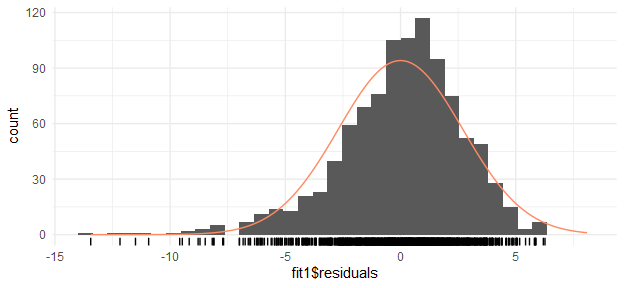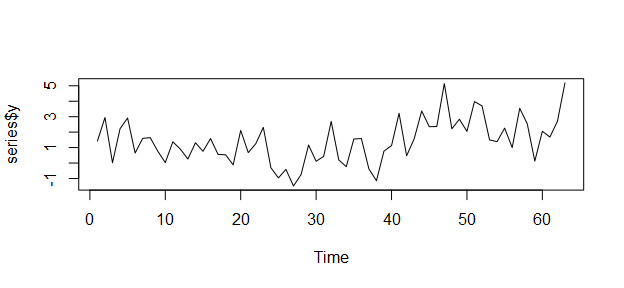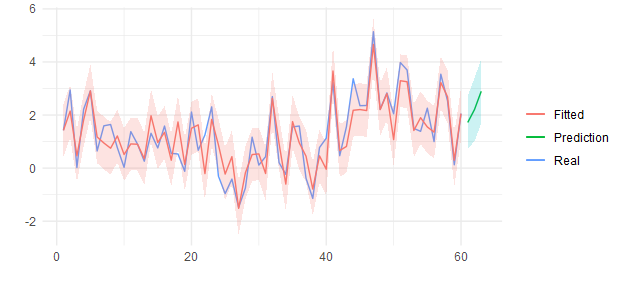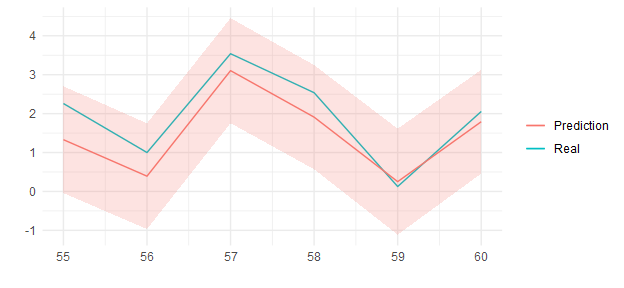# Using ConsReg Package

## Introduction

One of my main problems in my day-to-day work developing predictive models is in the logic of the model. When I talk about the model logic I refer that: the coefficients of the predictive variables make sense from an economic reasoning, the weight of each variable introduced is reasonable, the variables requested by business department have been incorporated,… and of course the error of the model is low!

Imagine the situation, you build a model with Decision Trees for the prediction of credit default: you get low train and test errors, you detect the variables that have more influence and these are from an economic logic point of view (income, savings, loan information, educational level,…), you prepare a shiny app so that the client can “play” with the model and can introduce some random values to the predictive variables,… everything is perfect until after a few days he calls you saying that he doesn’t understand why the model returns a much higher probability of default for a person with a doctorate degree than a person with a lower level of studies.

It could also happen, for example, that by slightly modifying the income level, the probability changes considerably, while by modifying the savings, it changes practically nothing.

These facts can be influenced by how the data are generated (risk policies followed by the company), for not having enought observations or by the structure of the data itself.

The methods that are usually used to correct are, for example, elimination directly of variables from the model that do not have the desired effect, processing of variables (combination of varialbes), regularization, Bayesian methods incorporating priors in the parameters (complicated, if you are not a Bayesian expert), … However, some of these methods do not solve your problem or you spend lots of time try to solve that.

Even if the model error is low, for a person not used to using predictive models, it is really difficult to trust a model that he does not understand and that the results it proposes are illogical. Sometimes, it can be convenient to sacrifice some error if you win in logic. And if the model is logical, it is more likely to generalize well in out-of-sample.

It is in the world of time series, in my experience, where this problem is most accentuated.

ConsReg is a collection of functions, that I have been writting and that I’ve put them together in this package, in order to estimate models with a priori constraints.

It has two main functions:

• ConsReg: to estimate linear and logistic regression models
• ConsRegArima: to estimate regression models with errors that follow an Arma process.

For the estimation of the parameters you can use functions from several specialized optimization packages. Even with MCMC simulation method optimization. I think the package is quite simple to use and intuitive. It is my first package I write in R and it is the first version of the package, so there will be many improvements! Please contact to me.

## Start

This first vignette is a first presentation of the package.

For the first example, I will use the fake_data dataset. This is a fake dataset only to show the functionalities of this package

require(ConsReg)
require(ggplot2)
require(data.table)

## ConsReg

data("fake_data")

fit1 = ConsReg(formula = y~x1+x2+x3+ I(x3^2) + x4, family = 'gaussian',
optimizer = 'solnp',
data = fake_data)
##
## Iter: 1 fn: 3.6337    Pars:  -1.651009  0.129054 -0.004316 -0.127660  0.020008  0.654738
## solnp--> Completed in 1 iterations
fit1$coefficients ## (Intercept) x1 x2 x3 I(x3^2) ## -1.651008854 0.129054342 -0.004316285 -0.127659853 0.020007889 ## x4 ## 0.654737554 coef(lm(y~x1+x2+x3+I(x3^2) + x4, data = fake_data)) ## (Intercept) x1 x2 x3 I(x3^2) ## -1.651008854 0.129054342 -0.004316285 -0.127659853 0.020007889 ## x4 ## 0.654737554 The use of the function ConsReg is very simple and similar to glm/lm function: • The formula term • family: a description of the error distribution: “gaussian” (linear regression), “binomial” for logistic regression or “poisson” (poisson regression) • optimizer: several optimizer functions from several packages are implmented. • data: data to be used Possible optimizers are: • solnp (default): Nonlinear optimization using augmented Lagrange method • gosolnp: Random Initialization and Multiple Restarts of the solnp solver • optim: General-purpose Optimization (stats package) • dfoptim: Hooke-Jeeves derivative-free minimization algorithm • nloptr: nloptr is an R interface to NLopt • DEoptim: Differential Evolution Optimization • mcmc: (from FME:ModMCMC) Constrained Markov Chain Monte Carlo • MCMCmetrop: random walk Metropolis algorithm • adaptMCMC: robust adaptive Metropolis sampler • GA: Genetic algorithms • GenSA: Generalized Simulated Annealing Function Additional arguments of each function can be passed to the function ConsReg. The object fit1 has the following information: Error metrics: fit1$metrics
LogLik RMSE MAE MAPE MSE SMAPE
-2410.638 2.695813 2.052224 4.255439 7.267408 1.086447

Residual analysis

forecast::gghistogram(fit1$residuals, add.normal = T, add.rug = T) + theme_minimal() ## Registered S3 method overwritten by 'xts': ## method from ## as.zoo.xts zoo ## Registered S3 method overwritten by 'quantmod': ## method from ## as.zoo.data.frame zoo ## Registered S3 methods overwritten by 'forecast': ## method from ## fitted.fracdiff fracdiff ## residuals.fracdiff fracdiffLet’s put some constraints to the model: • All coefficients will be less than 1 and greater than -1 • The coefficient of x3 and x3^2 must satisfied: (x3 + x3^2 > 0.01) • x4 < 0.2 It can be easily incoporate in the function: fit2 = ConsReg(formula = y~x1+x2+x3+ I(x3^2) + x4, data = fake_data, family = 'gaussian', constraints = '(x3 + I(x3^2)) > .01, x4 < .2', optimizer = 'mcmc', LOWER = -1, UPPER = 1, ini.pars.coef = c(-.4, .12, -.004, 0.1, 0.1, .15)) ## number of accepted runs: 893 out of 1000 (89.3%) To put in the function is just: • LOWER: -1 means that the lowest value that can take any coefficient is -1 • UPPER: 1 means that the highest value that can take any coefficient is 1 • constraints: is an string with the different restrictions in the coefficients. Each constraint must be separated by a comma. • ini.pars.coef: finally, this parameter is used to set the initial values. Those values must fulfill all the restrictions Observe that now, all coefficient fulfill our constraints: rbind(coef(fit1), coef(fit2)) ## (Intercept) x1 x2 x3 I(x3^2) x4 ## [1,] -1.6510089 0.1290543 -0.004316285 -0.1276599 0.02000789 0.6547376 ## [2,] -0.9731923 0.3265623 -0.011224336 0.3108502 -0.09635921 0.1972126 Also we can compare the errors to see that there is no much difference: rbind(fit1$metrics,
fit2$metrics) LogLik RMSE MAE MAPE MSE SMAPE -2410.638 2.695813 2.052224 4.255439 7.267408 1.086447 -2494.864 2.932706 2.152670 3.303897 8.600763 1.228690 For predictions, it follows the same system as a glm or lm object: pred = data.frame( fit1 = predict(fit1, newdata = fake_data[2:3,]), fit2 = predict(fit2, newdata = fake_data[2:3,]) ) pred fit1 fit2 2 -1.676538 -0.7073757 3 -2.263643 -1.6110852 Setting the parameter component = T, returns a matrix for the weight of each variable to the predictions pr = predict(fit2, components = T, newdata = fake_data[5,]) pr ## Total (Intercept) x1 x2 x3 I(x3^2) ## 5 -0.5119405 -0.9731923 0.4005724 0.01181268 0.9325506 -0.8672329 ## x4 ## 5 -0.01645103 ## ConsRegArima As I said, it is in the time series models where the problem mentioned above arises. In this case, a function has been implemented that estimates a regression with the Arima errors. This functions is quite similar to stats::arima function or in the forecast package, but the restrictions and constraints have been introduced. Also it can be write more friendly by using formula class. Let me show you. For this example, I will use another fake dataset data('series') The objective function has the following trend: plot(series$y, t='l')And the data set has 4 predictive variables:

head(series)
y x1 x2 x3 x4
1.4240582 -0.6051647 -0.2025728 0 -1.7669537
2.9434282 0.0531372 -1.9044371 0 -0.3468814
0.0227284 1.1121740 0.7198898 0 1.8846833
2.2191330 1.2894990 -1.7833160 0 0.1728917
2.9136307 -2.1313672 -2.1982599 0 -2.5840346
0.6459775 0.3245861 0.3002453 0 -0.7843327

We will estimate a first arma model (1, 1) with no regressors and no intercept

fit_ts1 = ConsRegArima(y ~ -1, order = c(1, 1), data = series[1:60, ])
##
## Iter: 1 fn: 0.1970    Pars:   0.97980 -0.72928
## Iter: 2 fn: 0.1970    Pars:   0.97980 -0.72928
## solnp--> Completed in 2 iterations
fit_ts1$coefficients ## ar1 ma1 ## 0.9798030 -0.7292797 coef(arima(series$y[1:60], order = c(1, 0, 1), include.mean = F, method = 'CSS'))
##        ar1        ma1
##  0.9798008 -0.7292652

Next I will add some regressors to the model:

fit_ts2 = ConsRegArima(y ~ x1+x2+x3+x4, order = c(1, 1), data = series[1:60,])
##
## Iter: 1 fn: -0.7311   Pars:   0.82849  0.39639 -0.80844  1.35773 -0.40255 -0.33677  1.16707
## Iter: 2 fn: -0.7311   Pars:   0.82849  0.39639 -0.80844  1.35773 -0.40255 -0.33677  1.16707
## solnp--> Completed in 2 iterations

Next I will add some constraints to the model:

fit_ts3 = ConsRegArima(y ~ x1+x2+x3+x4, order = c(1, 1), data = series[1:60,],
LOWER = -1, UPPER = 1,
constraints = "x4 < x2",
ini.pars.coef = c(.9, .3, -.1, .3, -.3),
control = list(trace = 0) #  not show the trace of the optimizer
)
fit_ts3$coefficients ## (Intercept) x1 x2 x3 x4 ar1 ## 0.9983209 0.2870705 -0.4862388 0.4804189 -0.4862388 -0.3641870 ## ma1 ## 0.6373165 To put in the function is just: • LOWER: -1 means that the lowest value that can take any coefficient is -1 • UPPER: 1 means that the highest value that can take any coefficient is 1 • constraints: is an string with the different restrictions in the coefficients. . • ini.pars.coef: finally, this parameter is used to set the initial values only for the regression coefficient. Those values must fulfill all the restrictions Next, we will change the optimizer. Let’s try with a genetic algorithm: fit_ts4 = ConsRegArima(y ~ x1+x2+x3+x4, order = c(1, 1), data = series[1:60,], LOWER = -1, UPPER = 1, constraints = "x4 < x2", penalty = 10000, optimizer = 'GA', maxiter = 1000, monitor = NULL, # not show the trace of the optimizer ini.pars.coef = c(.9, .2, 0, .3, -.6) ) fit_ts4$coefficients
## (Intercept)          x1          x2          x3          x4         ar1
##   0.8739497   0.4262889  -0.5202935   0.9974570  -0.5436331  -0.2806282
##         ma1
##   0.9539885

The restrictions are still fulfilled We can compare the errors of the 4 models:

data.frame(
metrics = colnames(fit_ts1$metrics), fit_ts1 = as.numeric(fit_ts1$metrics),
fit_ts2 = as.numeric(fit_ts2$metrics), fit_ts3 = as.numeric(fit_ts3$metrics),
fit_ts4 = as.numeric(fit_ts4$metrics) ) metrics fit_ts1 fit_ts2 fit_ts3 fit_ts4 ME 0.1176876 -0.0022704 0.1183575 0.0523606 RMSE 1.2075971 0.4773731 0.7518715 0.5971884 MAE 0.9814655 0.3849611 0.6203518 0.4842597 MPE -179.8131183 -24.5363218 -33.0289775 -52.0671108 MAPE 322.1692219 80.1435850 133.7496066 126.4981995 For predictions you will see that is very easy: pred = predict(fit_ts4, newdata = series[61:63, ], h=3, intervals = 90) pred$predict
Prediction Prediction_High Prediction_Low
61 1.720644 2.711354 0.7299348
62 2.208070 3.402294 1.0138447
63 2.895303 4.104100 1.6865051

And this object, you can plot to see the predictions as well as the fitted values:

plot(pred) + theme_minimal()In the ConsRegArima function, you can introduce the seasonal part P,Q, or in the formula, you can introduce lags in the predictor variables:

fit_ts5 = ConsRegArima(y ~ x1+x3+
shift(x3, 2) + # x2 from 2 periods above
x4,
order = c(1, 1), data = series[1:60,],
seasonal = list(order = c(1, 0), period = 4), # seasonal component
control = list(trace = 0)
)

If you have used lags in the predictive variables, then, in the predict function, you must add the original data:

pred = predict(fit_ts5, newdata = series[61:63,], origdata = series[1:60,])
pred$predict Prediction Prediction_High Prediction_Low 59 2.191149 3.601062 0.7812351 60 2.503279 4.006080 1.0004775 61 2.193245 3.706991 0.6794988 Finally, I have implemented a feature that I miss in many time series packages which is the possibility of backtesting. For a ConsRegArima object, I have implemented the “rolling” function that allows rolling-forecast with recalibration every $$n$$ periods, and projections to $$h$$ periods. And of course, very easy to carry out! ro = rolling(object = fit_ts3, used.sample = 50, refit = 4, h = 4, orig.data = series) In this case, the arguments are: • object: fit_ts3 • used.sample: sample to estimate the first refit. In this case we will use 1 to 50 observations • refit each 4 periods • predictions to 4rth period. The errors of the rolling are: And graphically: plot(ro) + theme_minimal()We can compare that errors of 4-step-forecasts are greater than 1-step-forecast: ro$results
xx Real Prediction Prediction_High Prediction_Low Fitted
55 2.2588072 1.3297014 2.702927 -0.0435246 1.3470754
56 0.9998795 0.3919824 1.750749 -0.9667838 0.6849166
57 3.5387356 3.1040633 4.453682 1.7544449 3.0891555
58 2.5365840 1.9089582 3.247440 0.5704760 2.0334505
59 0.1263169 0.2512654 1.612710 -1.1101792 0.3981201
60 2.0548210 1.7922128 3.124912 0.4595141 1.6842149